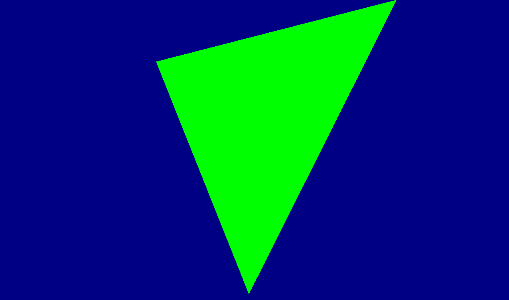## Push Constants

We can render arbitrary meshes now, but there is not a lot we can do with them. We still don’t know how to send data from the CPU to shaders outside of vertex buffers. While the next chapter will explain the various methods in detail, there is a simple method we can start using immediately: push constants.

Push constants let us send a small amount of data (it has a limited size) to the shader, in a very simple and performant way. Push constants can send data to any shader stage (both vertex and fragment shaders), and are stored in the command buffer itself.

To use push constants, you first need to set their size (in each stage) when you create a VkPipelineLayout. Then, using the command `vkCmdPushConstants`, the data will be embedded into the command buffer, and will be accessible from the shader. We are going to use them for transformation matrices, to be able to move objects around and do proper 3d rendering. To start, we are going to use it to move the triangle we are rendering.

## New PipelineLayout

We are going to need a new PipelineLayout to hold the push constant sizes. For that, we are going to add a new member to vulkanEngine.

``````class VulkanEngine {
public:
///other code...
VkPipelineLayout _meshPipelineLayout;
//other code ...

}
``````

We are also going to create a struct to hold it in `vk_engine.h`

``````//add the include for glm to get matrices
#include <glm/glm.hpp>

struct MeshPushConstants {
glm::vec4 data;
glm::mat4 render_matrix;
};
``````

For now, we will just store a single `glm::vec4` into it (4 floats) and a `glm::mat4` (16 floats). We will then use the matrix to transform the triangle. When you create push constant structs, alignment rules apply. You can find the exact rules for them by reading about the GLSL `std430` layout. These can be very complicated, so for simplicity, we are going to restrict ourselves to `glm::vec4` and `glm::mat4`, which have simple alignment rules. Push constants have a minimum size of 128 bytes, which is enough for common things like a 4x4 matrix and a few extra parameters.

To make space for the push constants in the pipeline, we create it inside the `init_pipelines()` function

``````
//we start from just the default empty pipeline layout info
VkPipelineLayoutCreateInfo mesh_pipeline_layout_info = vkinit::pipeline_layout_create_info();

//setup push constants
VkPushConstantRange push_constant;
//this push constant range starts at the beginning
push_constant.offset = 0;
//this push constant range takes up the size of a MeshPushConstants struct
push_constant.size = sizeof(MeshPushConstants);
//this push constant range is accessible only in the vertex shader

mesh_pipeline_layout_info.pPushConstantRanges = &push_constant;
mesh_pipeline_layout_info.pushConstantRangeCount = 1;

VK_CHECK(vkCreatePipelineLayout(_device, &mesh_pipeline_layout_info, nullptr, &_meshPipelineLayout));

//later ....
//remember to destroy the pipeline layout
_mainDeletionQueue.push_function([=]() {
//other deletions

vkDestroyPipelineLayout(_device, _meshPipelineLayout, nullptr);
});

``````

Push constants are written in ranges. A important reason for that, is that you can have different push constants, at different ranges, in different stages. For example, you can reserve 64 bytes (1 `glm::mat4`) size on the vertex shader, and then start the pixel shader push constant from offset 64. This way you would have different push constants on different stages. Given that getting the ranges right is tricky, in this tutorial we are going to use a more monolithic approach of using the same push constants on both vertex and fragment shader.

Now that we have the layout, we also need to modify the shaders. Let’s add the push constant to the `tri_mesh.vert` shader.

``````
#version 450

layout (location = 0) in vec3 vPosition;
layout (location = 1) in vec3 vNormal;
layout (location = 2) in vec3 vColor;

layout (location = 0) out vec3 outColor;

//push constants block
layout( push_constant ) uniform constants
{
vec4 data;
mat4 render_matrix;
} PushConstants;

void main()
{
gl_Position = PushConstants.render_matrix * vec4(vPosition, 1.0f);
outColor = vColor;
}
``````

The push_constant block has to match to the struct we have on C++ side 1 to 1, otherwise the GPU will read our data incorrectly.

Now we can modify the way we create the triangle pipeline to hook the new pipeline layout.

in `init_pipelines`, right before creating the mesh pipeline, we are going to hook the new layout

``````
pipelineBuilder._pipelineLayout = _meshPipelineLayout;

_meshPipeline = pipelineBuilder.build_pipeline(_device, _renderPass);
``````

Now our mesh pipeline has the space for the push constants, so we can now execute the command to use them.

in `draw()` function, right before the `vkCmdDraw` call, we are going to compute and write the push constant.

``````    //make a model view matrix for rendering the object
//camera position
glm::vec3 camPos = { 0.f,0.f,-2.f };

glm::mat4 view = glm::translate(glm::mat4(1.f), camPos);
//camera projection
glm::mat4 projection = glm::perspective(glm::radians(70.f), 1700.f / 900.f, 0.1f, 200.0f);
projection *= -1;
//model rotation
glm::mat4 model = glm::rotate(glm::mat4{ 1.0f }, glm::radians(_frameNumber * 0.4f), glm::vec3(0, 1, 0));

//calculate final mesh matrix
glm::mat4 mesh_matrix = projection * view * model;

MeshPushConstants constants;
constants.render_matrix = mesh_matrix;

//upload the matrix to the GPU via push constants
vkCmdPushConstants(cmd, _meshPipelineLayout, VK_SHADER_STAGE_VERTEX_BIT, 0, sizeof(MeshPushConstants), &constants);

//we can now draw
vkCmdDraw(cmd, _triangleMesh._vertices.size(), 1, 0, 0);

``````

You will also need to add

``````#include <glm/gtx/transform.hpp>
``````

To the `vk_engine.cpp` file so that you can use the glm transformation matrix functions.

In the push constant call, we need to set the pointer to the data and its size (similar to `memcpy`), and also `VK_PIPELINE_STAGE_VERTEX_BIT`, which matches the stage(s) where our data should be accessible. If we have the same push constant on both vertex and fragment shader, we would have both of those flags.

If you now run the program, you will see the triangle spinning in the window, upside down. By modifying `camPos` and the view matrix in general, you can now create a 3d camera.